# IDA

NLA
QRSlv
CMSlv
IPSlv
NLP
CONOPT
IPOPT
TRON
MINOS
Opt
NGSlv
DAE/ODE
IDA
LSODE
DOPRI5
LA
Linsolqr
Linsol
LP
MakeMPS
Logic
LRSlv

IDA is a differential-algebraic equation (DAE) integrator. by Alan Hindmarsh, Radu Serban, and others, from LLNL. It provides multi-step implicit DAE integration techniques using backwards difference formulae and variable time-steps.

This means that it can be used to solve dynamic problems where there are equations containing time derivatives, plus optionally algebraic equations that provide 'constraint' behaviour.

The general form of problems that IDA can solve is a DAE system,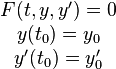$\begin{matrix} F(t,y,y') = 0 \\ y(t_0)= y_0 \\ y'(t_0) = y'_0 \end{matrix}$

where y and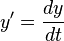$y'=\frac{dy}{dt}$are vectors in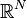$\R^N$ and t is the independent variable, and the solution y(t) is sought for t > t0.

The syntax for writing such equations with ASCEND is something that we're working on. See DynamicModelling for the status quo.

## Partitioned form

For the purpose of understanding how ASCEND deals with systems in order that they can be solved with IDA, it is useful to define the partitioned form of the DAE. For this form, we divide up the variables into three sets:

•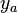${y}_a$ - the algebraic variables: those variables for which no derivative is present
•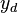${y}_d$ - the differential variables: those for which a derivative is present
•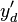$y'_d$ - the derivative variables: derivatives of$y_d$

Then it is simple to also divide up the set of equations in to two sets:

•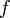${f}$ - the differential equations: those which include one or more derivative variables
•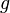${g}$ - the algebraic equations: those which include no derivative variables

The system can then be written$\begin{matrix} f(t,y'_d,y_a,y_d) & = & 0 \\ g(t,y_a,y_d) & = & 0 \end{matrix}$

The initial conditions are as before. In the above it can be seen that the system has a set of equations without any derivatives at all. This form is also called ODEs with constraints and is the split made by ASCEND when the LSODE solver is applied to a general DAE problem.

IDA has the ability to export the matrices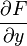$\frac{\partial F}{\partial y}$ and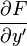$\frac{\partial F}{\partial y'}$ but at present can not be used to compute the matrix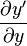$\frac{\partial y'}{\partial y}$ directly (which LSODE can do). See also MatrixMarket.

## Initial conditions

In a DAE system, there are more variables than equations so initial conditions require some additional information before they can be solved. You have the choice of putting the model into a consistent starting state yourself, or else you can use IDA to solve for the initial conditions in two different scenarios, according the the value of the solver parameter calcic:

1. calcic='Y''. Use the values of the derivatives y(t0) to solve for all the non-derivatives y(t0). This is useful for 'steady' initial conditions, for example, where all the derivatives are set to zero.
2. calcic='YA_YDP'. Use the values of the differential variables yd(t0) to solve for the derivatives yd'(t0) and the algebraic variables ya(t0). This is useful when you want to 'release' the system from a known (perhaps off-equilibrium) state and watch how it evolves. This is also useful for continuing a simulation after a discontinuity.

## Linear solvers

IDA (as shipped by LLNL) has the ability to accommodate various linear solvers (the linsolver option) including both direct and iterative (Krylov) solvers.

In ASCEND, access to the Krylov SPGMR solver is implemented, but there is as yet no sensible approach for the preconditioner, so it's not particularly useful.

We also have implemented access to the DENSE direct solver. This works well, but obviously shouldn't be used on very large models (limit seems to be about 200-400 variables on a typical desktop machine)

IDA provides a banded direct solver but support for this is not implemented.

Values of linsolver include (check the GUI for the current list):

SPGMR
DENSE


We also plan to add support (as linsolver='ASCEND')for our own sparse direct solver (linsolqr) but this is not yet complete. Alternatively we might connect another linear solver such as UMFPACK, KLU or SuperLU.

## Index problems

It's very common when simulating any non-trivial physical system to come across index problems, and it's important to understand how these can be overcome. There are automated index-reduction tools offered by some simulation environments, but ASCEND does not currently provide this.

For index problems not to exist, one test is to FIX the differential variables (yd) and then to calculate the system Jacobian for the remaining NLA system, d'F / d'y * where y * = [ya | yd']. This this matrix is singular, there is an index problem<a href="#_note-art" title=""></a>

Alternatively one can compute d'g / d'ya and if that matrix is non-singular, the index of the system is index 1. This applies for cases where the system is semi-implict -- not sure if it applies generally to any system you write with ASCEND therefore.

This second approach is currently implemented in the IDA solver's integrator_ida_analyse. The routine simply extracts the d'g / d'ya matrix from the system and uses linsolqr to evaluate the matrix rank (providing the matrix exists -- if it does not, the you have a system of ODEs). If the matrix is full-rank, then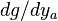$dg/dy_a$ is non-singular, and the system is of index 1, so IDA should not have any index problem.

In general it seems that we might also need to check that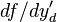$df/d{y_d'}$ is non-singular?

## Stability

Compared to a pure ODE system, it takes some work to determine the stability of a DAE system. If we write the system in the f,g form, we can procede by<a href="#_note-art" title=""></a>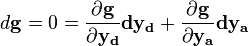$d\mathbf{g} = 0 = \frac{\partial \mathbf{g}}{\partial \mathbf{y_d}}\mathbf{dy_d} + \frac{\partial \mathbf{g}}{\partial \mathbf{y_a}}\mathbf{dy_a}$

and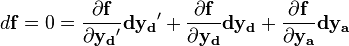$d\mathbf{f} = 0 = \frac{\partial \mathbf{f}}{\partial \mathbf{y_d}'}\mathbf{dy_d}' + \frac{\partial \mathbf{f}}{\partial \mathbf{y_d}}\mathbf{dy_d} + \frac{\partial \mathbf{f}}{\partial \mathbf{y_a}}\mathbf{dy_a}$

We set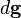$d\mathbf{g}$ and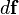$d\mathbf{f}$ to zero, and seek to eliminate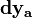$\mathbf{dy_a}$ from the equations: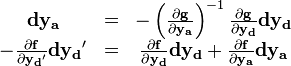$\begin{matrix} \mathbf{dy_a} & = & - \left ( \frac{\partial \mathbf{g}}{\partial \mathbf{y_a}} \right )^{-1} \frac{\partial \mathbf{g}}{\partial \mathbf{y_d}}\mathbf{dy_d} \\ -\frac{\partial \mathbf{f}}{\partial \mathbf{y_d}'}\mathbf{dy_d}' & = & \frac{\partial \mathbf{f}}{\partial \mathbf{y_d}}\mathbf{dy_d} + \frac{\partial \mathbf{f}}{\partial \mathbf{y_a}}\mathbf{dy_a} \end{matrix}$

Then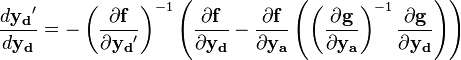$\frac{d\mathbf{y_d}'}{d\mathbf{y_d}} = - \left (\frac{\partial \mathbf{f}}{\partial \mathbf{y_d}'}\right)^{-1} \left ( \frac{\partial \mathbf{f}}{\partial \mathbf{y_d}} - \frac{\partial \mathbf{f}}{\partial \mathbf{y_a}}\left (\left ( \frac{\partial \mathbf{g}}{\partial \mathbf{y_a}} \right )^{-1} \frac{\partial \mathbf{g}}{\partial \mathbf{y_d}} \right) \right)$

The eigenvalues of this matrix (real and imaginary) determine the system stability.

You can fairly easily plot these eigenvalues now using ASCEND. See Damped response for a very simple example.

## Root-finding

IDA provides functionality for boundary-crossing detection (root-finding). Currently, ASCEND will detect boundaries declared in your model using the CONDITIONAL statement, and will set IDA to watch for when those boundaries are crossed. The remaining parts of the ASCEND conditional modelling functionality are not yet implemented however. This would require that when a boundary is crossed, ASCEND check for affected WHEN statements, and if any are found reconfigure the model, remove certain equations and add other ones as necessary. IDA would then need to 'restart' the integration, which effectively means solving the initial condition problem again and then continuing the integration.

## Solver parameters

The documentation for these parameters is available via the PyGTK interface (the Integrator dialog) or via the source code for solvers/ida/ida.c. This list is still incomplete so the documentation won't be added here just yet.

calcic
linsolver
maxl
autodiff
safeeval
rtol
atol
atolvect
gsmodified
maxncf
prec


## Internal data structure

Variables. The diagram at right shows the internal data structure used to track variables in DAE models with IDA.

The slv_get_solvers_var_list(integ->system) contains all the variables including those that not involved in the system.

The list integ->y (called 'blsys->y' in the diagram) contains all the non-derivative variables. The differential variables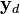$\mathbf{y}_d$ and the algebraic variables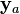$\mathbf{y}_a$ are all mixed up together (so that block partitioning can be used in future). Differential variables can be identified by the fact that integ->ydot[var_sindex[var]] will be non-NULL.

A variable can be identified as a derivative using the VAR_DERIV flag (or the var_deriv(var) function) or by seeing that its var_sindex(var) is greater than integ->n_y (it has been moved after all the non-derivative variables).

To obtain the differential variable corresponding to a derivative variable, use the integrator_ida_diffindex(var) function.

The number of derivatives (and hence number of differential variables) is integ->n_ydot. The number of algebraic variables is integ->n_y - integ->n_ydot.

Equations are also partitioned. Relations are flagged with the REL_DIFFERENTIAL flag if they were found to include derivatives. This enables easy iteration through either differential or algebraic equations. And the number of differential equations is stored in integ->n_diffeqs.

Note that previously 'blsys' or 'sys' were used instead of the new preferred variable name 'integ'.

See also IDA/Analysis for some more details on the algorithm that ASCEND uses to prepare a model for integration with IDA.

## Example problems

Check the following files in the ModelLibrary

johnpye/datareader/testtmy.a4c
johnpye/lotka.a4c
johnpye/newton.a4c
johnpye/thermalequilibrium2.a4c
johnpye/shm.a4c
test/hires.a4c
test/pollution.a4c
test/chemakzo.a4c
test/transamp.a4c
twinslabs.a4c


For more thrills, try find models -name "*.a4c" | xargs grep -n "\"ivpsystem.a4l"

## FAQ

Can I plot my results?

Yes! You can plot your results, as of version 0.9.5.112. You need to have Matplotlib installed on your machine. The 'Integrator' tab will then include a 'plot' button that will plot the second column of results against the first column. You can also plot other stuff, using Console commands. See Plotting in ASCEND for more details.

Alternatively, it is also quite easy to use the PyGTK interface to copy the results from the 'Integration' tab (press ctrl-C) then paste into OpenOffice or Excel. Then you can plot your results there, with a little more ease, possibly.

How do I build IDA on Windows?